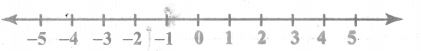You can Download Samacheer Kalvi 6th Maths Book Solutions Guide Pdf, Tamilnadu State Board help you to revise the complete Syllabus and score more marks in your examinations.## Tamilnadu Samacheer Kalvi 6th Maths Solutions Term 3 Chapter 2 Integers Ex 2.1

Question 1.
Fill in the blanks.
(i) The potable water available at 100m below the ground level is denoted as ______ m.
Hint: Below ground level – negative; ground level – 0; above ground level – positive.
(ii) A swimmer dives to a depth of 7 feet from the ground into the swimming pool. The integer that represents this, is ______ feet.
Hint: Below ground level – negative numbers.
(iii) -46 is to the _____ of -35 on the number line.
Hint: -46 <-35
(iv) There are _____ integers from -5 to +5 (both inclusive).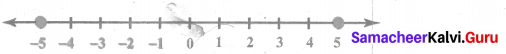(v) ______ is an integer which is neither positive nor negative.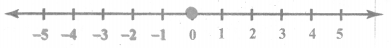Solutions:
(i) -100
(ii) -7
(iii) Left
(iv) 11
(v) 0

Question 2.
Say True or False.
(i) Each of the integers -18, 6, -12, 0 is greater than -20.
(ii) -1 is to the right of 0.
(iii) -10 and 10 are at equal distance from 1.
(iv) All negative integers are greater than zero.
(v) All whole numbers are integers.
Solution:
(i) True
(ii) False
(iii) False
(iv) False
(v) True

Question 3.
Mark the numbers 4, -3, 6, -1 and -5 on the number line.
Solution: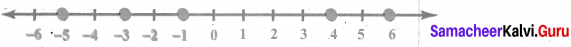Question 4.
On the number line, which number is
i) 4 units to the right of-7?
ii) 5 units to the left of 3?
Solution: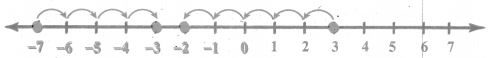(i) – 3 is 4 units to the right of -7
(ii) – 2 is 5 units to the left of 3Question 5.
Find the opposite of the following numbers.
(i) 44
(ii) -19
(iii) 0
(iv) -312
(v) 789
Solution:
(i) Opposite of 44 is – 44
(ii) Opposite of-19 is + 19 or 19
(iii) Opposite of 0 is 0
(iv) Opposite of-312 is + 312 or 312
(v) Opposite of 789 is – 789.

Question 6.
If 15 km east of a place is denoted as + 15 km, what is the integer that represents 15 km west of it?
Solution:
Opposite of east is west.
∴ If 15 km east is + 15 km, then 15 km west is – 15 km.

Question 7.
From the following number lines, identify the correct and the wrong representations with reason?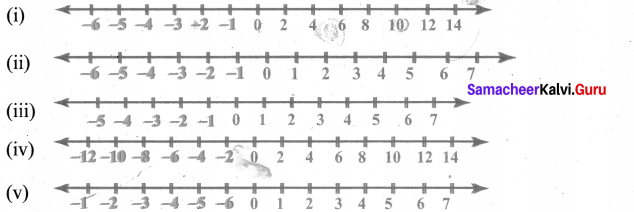Solution:
(i) This representation is wrong. Because the numbers are not continuously marked as -6, -5, -4, -3, -2, -1, 0, 1, 2, 3, 4, 5, 6,
(ii) This is the correct representation of Integers
(iii) The representation is wrong. – 2 is misplaced in the number line.
(iv) This representation is correct as the numbers are marked at equal intervals in the correct order.
(v) Here negative integers are marked wrongly to the left of 0

Question 8.
Write all the integers between the given numbers.
(a) 7 and 10
(b) -5 and 4
(c) -3 and 3
(d) -5 and 0
Solution: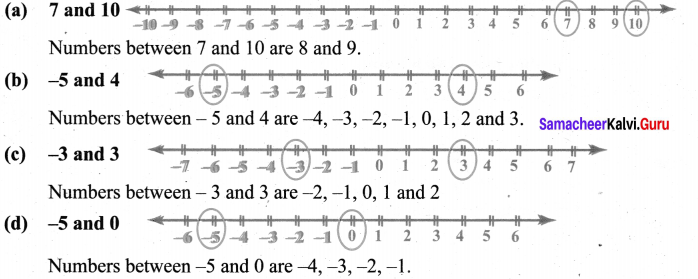Question 9.
Put the appropriate signs as <, > or = in the box?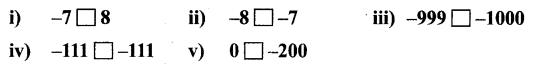Solution:
(i) We know that -7 is to the left of 8.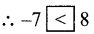(ii) -8 is to the left of -7,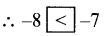(iii) -1000 is to the left of -999,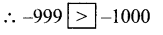(iv) -111 and-111 coincides in the number line.(v) 0 is to the right of -200,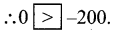Question 10.
Arrange the following integers in ascending order.
i) -11, 12, -13, 14, -15, 16, -17, 18, -19, -20
ii) -28, 6, -5, -40, 8, 0, 12, -1, 4, 22
iii) -100, 10, -1000, 100,0, -1, 1000, 1, -10
Solution:
(i) -11, 12, -13, 14, -15, 16, -17, 18, -19, -20

• First separating the positive integers 12, 14, 16, 18 and the negative integers -11,-13,-15,-17,-19,-20.
• Then arranging the positive integers in ascending order we get 12, 14, 16, 18 and negative integers in ascending order as -20, -19, -17, -15, -13, -11 4
• Now the ascending order : -20, -19, -17, -15, -13, -11, 12, 14, 16, 18.

(ii) -28, 6, -5, -40, 8, 0, 12, -1, 4, 22

• Positive integers are 6, 8, 12, 4, 22 Negative integers are -28, -5, -40, -1
• Arranging the positive integers in ascending order we get 4, 6, 8, 12, 22 and the negative integers in ascending order -40, -28, -5, -1
• The number 0 is neither positive nor negative and stand in the middle.
• In ascending order : -40, -28, -5, -1, 0, 4, 6, 8,12, 22

(iii) -100, 10, -1000, 100, 0, -1, 1000, 1, -10

• Separating positive integers 10, 100, 1000, 1 and negative integers -100, -1000, -1, -10.
• Now the positive integers in ascending order 1,10,100,1000 and the negative integers in ascending order. -1000, -100, -10, -1
• Also ‘0’ stand in the middle as its is neither positive nor negative.
• ∴ The numbers in ascending order: -1000, -100, -10, -1, 0, 1, 10, 100, 1000.Question 11.
Arrange the following integers in descending order.
i) 14, 27, 15, -14, -9, 0, 11, -17
ii) -99, -120, 65, -46, 78, 400, -600
iii) 111, -222, 333, -444, 555, -666, 7777, -888
Solution:
(i) 14, 27, 15, -14, -9, 0,11, -17

• Separating the positive integers 14, 27, 15, 11 and negative integers -14, -9, -17
• Arranging in descending order we get the positive integers 27,15,14,11 and the negative integers -9, -14, -17.
• ‘0’ is neither positive nor negative and so it stand in middle.
• ∴ The numbers in descending order : 27, 15, 14, 11, 0, -9, -14, -17

(ii) -99, -120, 65, -46, 78, 400, -600

• Separating the positive integers 65, 78, 400 and negative integers -99, -120, -46, -600
• Arranging the positive integers in descending order as 400, 78, 65 and the negative integers in descending order -46, -99, -120, -600.
• The numbers in descending order : 400, 78, 65, -46, -99, -120, -600.

(iii) 111, -222, 333, -444, 555, -666, 7777, -888

• Separating the positive integers 111, 333, 555, 7777 and negative integers -222, -444, -666, -888
• Arranging the positive integers in descending order as 7777, 555, 333, 111 and negative integers in descending order as -222, -444, -666, -888
• The numbers in descending order : 7777, 555, 333, 111, -222, -444, -666, -888

Objective Type Questions

Question 12.
There are _______ positive integers from – 5 to 6.
(a) 5
(b) 6
(c) 7
(d) 11
Solution:
(b) 6
Hint: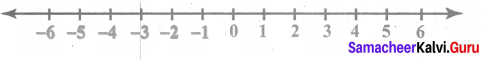Question 13.
The opposite of 20 units to the left of 0 is
(a) 20
(b) 0
(c) -20
(d) 40
Solution:
(a) 20
Hint: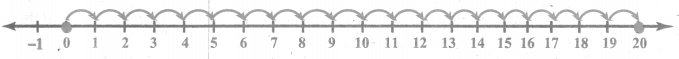Question 14.
One unit to the right of -7 is
(a) +1
(b) -8
(c) -7
(d) -6
Solution:
(d) -6
Hint: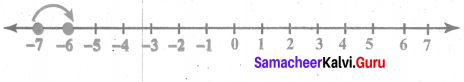Question 15.
3 units to the left of 1 is
(a) -4
(b) -3
(c) -2
(d) 3
Solution:
(c) -2
Hint: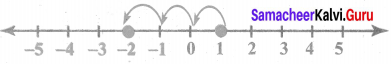Question 16.
The number which determines marking the position of any number to its opposite on a number line is?
(a) -1
(b) 0
(c) 1
(d) 10
Solution:
(b) 0
Hint: